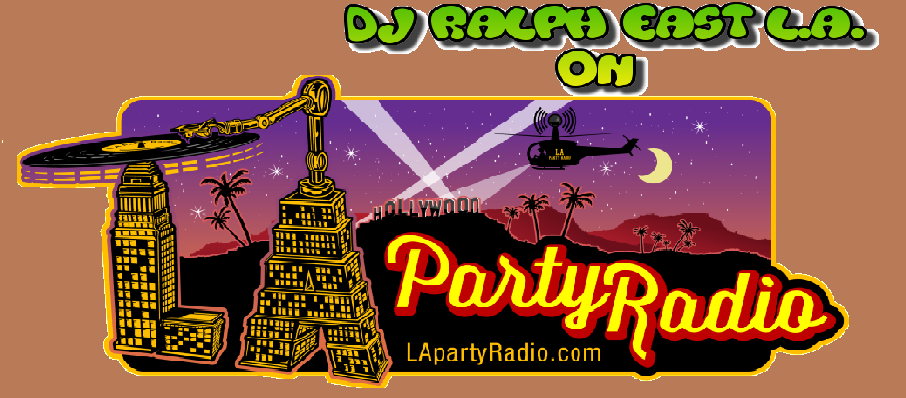[ Post a Response | Ultimate Crave Message Board ]

)))))))))))))))))))))))))))))))DJ RALPH EAST L.A. MIX!!!! ((((((((((((((((((((((((((((((by request one of my mixes feature on L.A.Party Radio.Com CHANTES ADVENTURE Radio Show every Friday get ready Friday night will never be the same, from 5 p.m. to 8 o'clock every Friday, now check out my mix click on the link---> https://soundcloud.com/djralph1980/dj-ralph-e-l-a-old-school-myMessage Thread )))))))))))))))))))))))))))))))DJ RALPH EAST L.A. MIX!!!! (((((((((((((((((((((((((((((( - DJ RALPH E.L.A. April 16, 2015, 1:28 am« Back to index

Post a Response